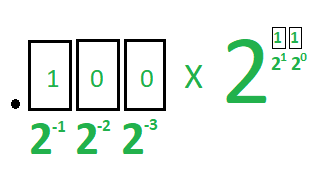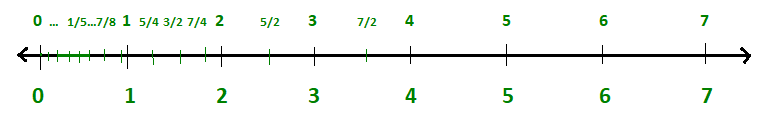# Why floating-point values do not represent exact value

The floating-point numbers serve as rough approximations of mathematical real numbers. They do not represent the exact value. For this reason, we compare the arithmetic results of float variables with a minimum tolerance value.
Example:

## C++

 // C++ program to illustrate the // floating point values #include  using namespace std;   // Driver Code int main() {     double num1 = 10000.29;     double num2 = 10000.2;       // Output should be 0.0900000000     cout << std::setprecision(15)          << (num1 - num2);     return 0; }

## Java

 // Java program to illustrate the // floating point values import java.text.DecimalFormat;   class GFG{   // Driver Code public static void main(String[] args) {     double num1 = 10000.29;     double num2 = 10000.2;       // Output should be 0.0900000000     DecimalFormat df = new DecimalFormat(         "#.################");               System.out.println(df.format(num1 - num2)); } }   // This code is contributed by 29AjayKumar

## Python3

 # Python3 program to illustrate  # the floating povalues # Driver Code if __name__ == '__main__':     num1 = 10000.29;     num2 = 10000.2;       # Output should be 0.0900000000     print ("{0:.10f}".format(num1 - num2));   # This code is contributed by Rajput-Ji

## C#

 // C# program to illustrate the // floating point values using System;   class GFG{   // Driver Code public static void Main(String[] args) {     double num1 = 10000.29;     double num2 = 10000.2;       // Output should be 0.0900000000     Console.WriteLine(         string.Format("{0:F15}",          Decimal.Parse((num1 - num2).ToString()))); } }   // This code is contributed by 29AjayKumar

Output:

0.0900000000001455



Explanation:
The expected output is 0.09 as output. But, the output is not 0.09. To understand this, you first have to know how a computer works with float values. When a float variable is initialized, the computer treats it as an exponential value and allocates 4 bytes(32 bits) memory where the mantissa part occupies 24 bits, the exponent part occupies 7 bits, and the remaining 1 bit is used to denote sign.
For type double, the computer does the same but allocates larger memory compared to the float type. In the decimal system, every position from(left to right) in the fractional part is one-tenth of the position to its left. If we move from right to left then every position is 10 times the position to its right.
In a binary system, the factor is two as shown in the table:To simplify things, let us think of a mythical type named small float(see the above image) which consists of only 5 bits – very small compared to float and double. The first three bits of the type small float will represent mantissa, the last 2 bits will represent the exponent part. For the sake of simplicity, we do not think about the sign. So the mantissa part can have only 8 possible values and the exponent part can only have 4 possible values. See the tables below:

So, one combination of mantissa and exponent part can be 11100 where the leftmost two bits represent the exponent part and the remaining three bits represent the mantissa part. The value is calculated as:From the two tables, we can easily say that a small float can contain only 32 numbers and the range of the mythical type is 0 to 7. The range is not equally dense. If you see the following image carefully you will see most values lie between 0 and 1. The more you move from right to left the more sparse the numbers will be.The small float can not represent 1.3, 2.4, 5.6, etc. In that case, small float approximates them. It can not represent numbers bigger than 7. Besides many combinations represent the same value. For example: 00000, 00001, 00010, 00011 represent the same decimal value i.e., (0.000). Twelve of the 32 combinations are redundant.
If we increase the number of bits allocated for small float, the denser portion will increase. As float values reserve 32 bits, float value can represent more numbers compared to small float. But some issues can be observed with float values and double values. There is no path to overcome this. Computers with infinite memory and fast preprocessor can only compute exact float or double values which is a fantasy for us.

My Personal Notes arrow_drop_upCheck out this Author's contributed articles.

If you like GeeksforGeeks and would like to contribute, you can also write an article using contribute.geeksforgeeks.org or mail your article to contribute@geeksforgeeks.org. See your article appearing on the GeeksforGeeks main page and help other Geeks.

Please Improve this article if you find anything incorrect by clicking on the "Improve Article" button below.

Improved By : 29AjayKumar, Rajput-Ji

Article Tags :

1

Please write to us at contribute@geeksforgeeks.org to report any issue with the above content.